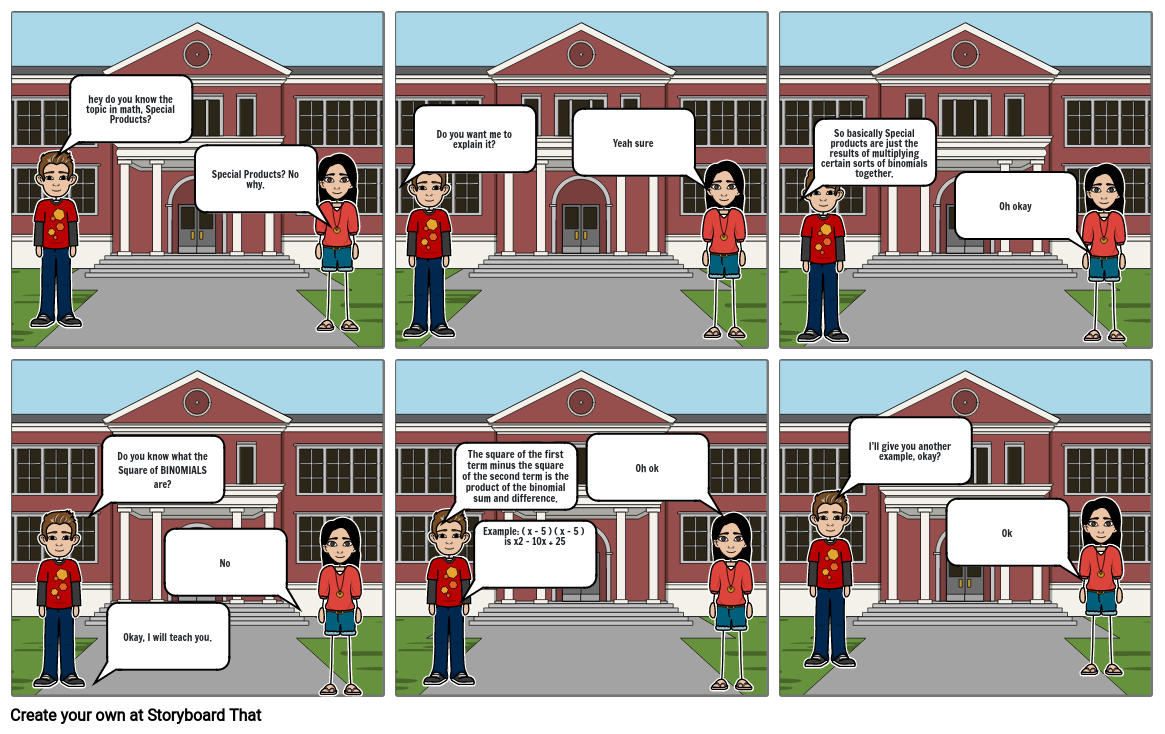Unknown StoryStoryboard Text

• hey do you know the topic in math, Special Products?
• ﻿Special Products? No why.﻿﻿
• Do you want me to explain it?﻿
• Yeah sure
• So basically Special products are just the results of multiplying certain sorts of binomials together.
• ﻿﻿﻿you know what binomials are right?
• Oh okay
• ﻿So basically Special products are just the results of multiplying certain sorts of binomials together. ﻿﻿
• Do you know what the Square of BINOMIALS are?
• ﻿Okay, I will teach you.
• ﻿No
• The square of the first term minus the square of the second term is the product of the binomial sum and difference.
• ﻿Example: ( x - 5 ) ( x - 5 ) is x2 - 10x + 25
• Oh ok
• I’ll give you another example, okay?
• Ok
• ﻿﻿
• ﻿﻿﻿= 9
Over 20 Million Storyboards Created